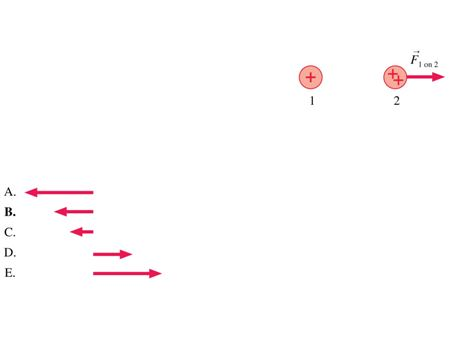# Problem: The charge of sphere 2 is twice that of sphere 1. Which vector below shows the force of 2 on 1?

###### FREE Expert Solution

From newton's third law of motion, every action has a reaction of equal magnitude, but in opposite direction.

95% (143 ratings)###### Problem Details

The charge of sphere 2 is twice that of sphere 1. Which vector below shows the force of 2 on 1?